Next: Matrix eigenvalue theory Up: Useful mathematics Previous: Conic sections

# Elliptic expansions

The well-known Bessel functions of the first kind,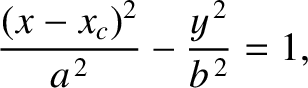, where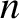is an integer, are defined as the Fourier coefficients in the expansion of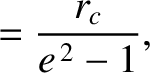: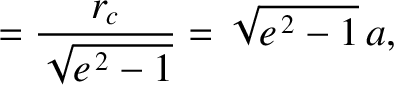(A.116)

It follows that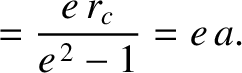(A.117)

(Gradshteyn and Ryzhik 1980a). The Taylor expansion ofabout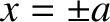is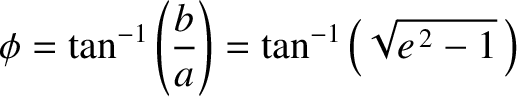(A.118)

for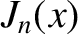(Gradshteyn and Ryzhik 1980b). Moreover,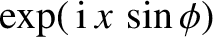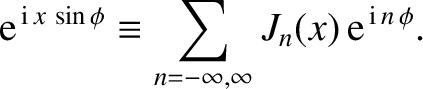(A.119) and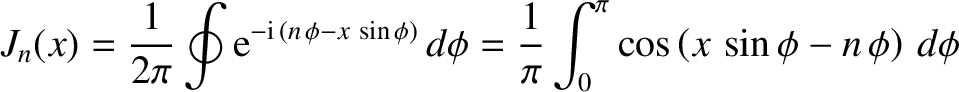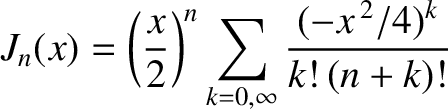(A.120)

In particular,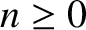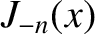(A.121)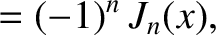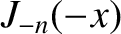(A.122)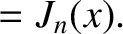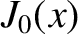(A.123) and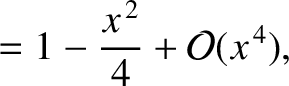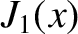(A.124)

Let us write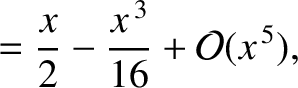(A.125)

whereis the eccentric anomaly, and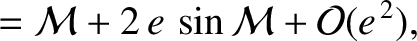the mean anomaly, of a Keplerian elliptic orbit. (See Section 4.11.) It follows that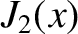(A.126)

Integrating by parts, we obtain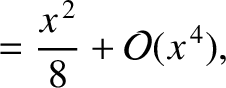(A.127)

However, according to Equation (4.59), the relationship between the eccentric and the mean anomalies is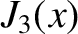(A.128)

where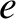is the orbital eccentricity. Hence,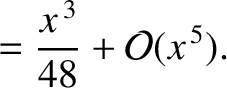(A.129)

Comparison with Equation (A.117) reveals that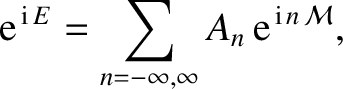(A.130)

For the special case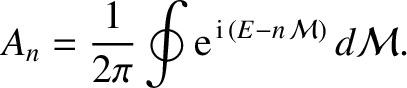, L'Hopital's rule, together with Equations (A.119) and (A.122), yields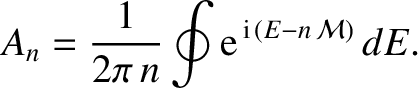(A.131)

where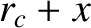denotes a derivative.

The real part of Equation (A.125) gives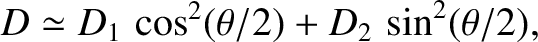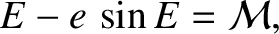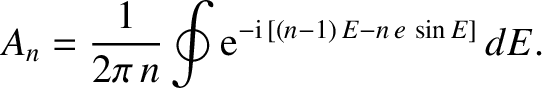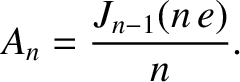(A.132)

where use has been made of Equations (A.120), (A.130), and (A.131). Likewise, the imaginary part of Equation (A.125) yields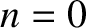(A.133)

It follows from Equations (A.121)-(A.124) that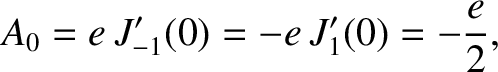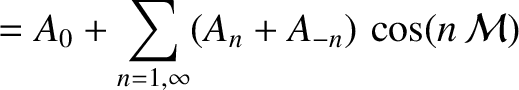(A.134) and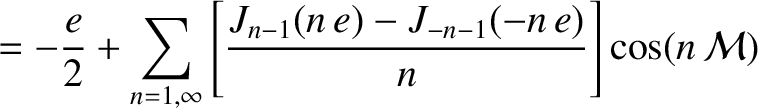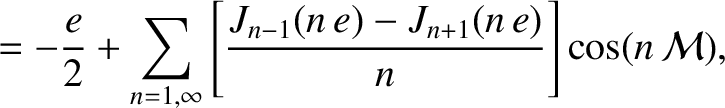(A.135)

Hence, from Equation (A.128),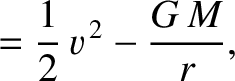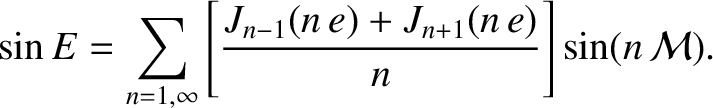(A.136)

According to Equation (4.69),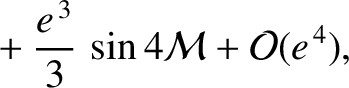(A.137)

where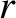is is the radial distance from the focus of the orbit andis the orbital major radius. Thus,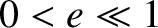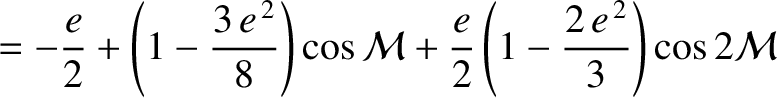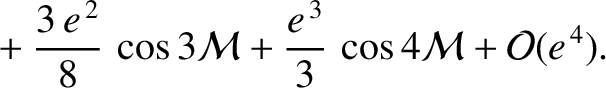(A.138)

Equations (4.39) and (4.67) imply that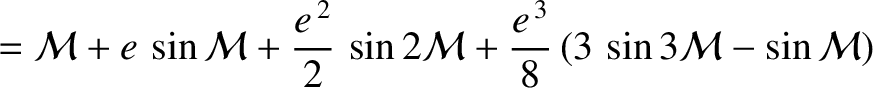(A.139)

where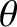is the true anomaly. Hence, it follows from Equations (A.128) and (A.137), and the fact that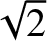when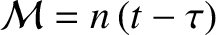, that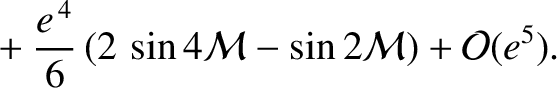(A.140)

From Equation (A.136),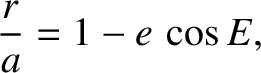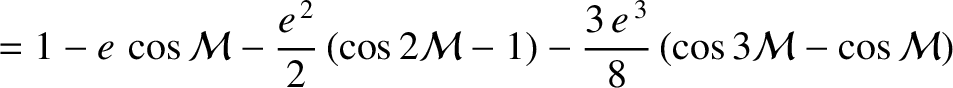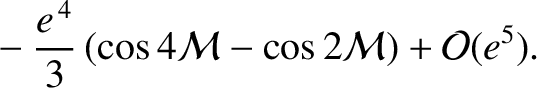(A.141)

Thus,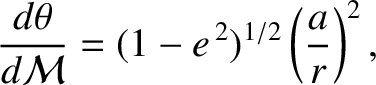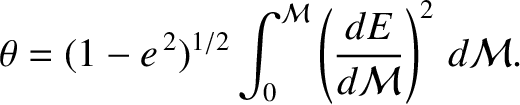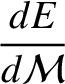(A.142)

and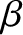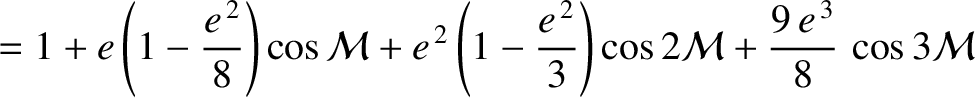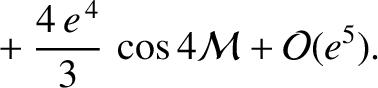(A.143)Next: Matrix eigenvalue theory Up: Useful mathematics Previous: Conic sections
Richard Fitzpatrick 2016-03-31